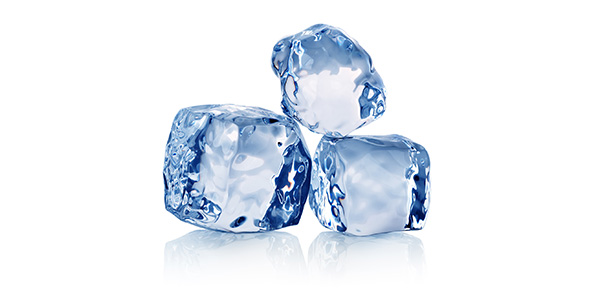# Glaciers

12 Questions | Total Attempts: 220SettingsA test to evaluate your current knowledge of the world of glaciers. This simply includes: deposits, formations, budgets, and more.

• 1.
What feature can be formed where a tributary glacier flows into a main/trunk glacier?
• A.

Horn

• B.

Arete

• C.

Terminal morraine

• D.

Hanging trough

• 2.
Iceberg ___ from their parent glacier.
• A.

Melt

• B.

Break

• C.

Clave

• D.

Calve

• 3.
What is the area above the snowline called?
• A.

Zone of wastage

• B.

Zone of accumulation

• C.

Norther hemisphere

• D.

Latitude

• 4.
In polar regions, how would you best describe polar regions?
• A.

Low altitudes

• B.

High altitudes

• C.

Below the snowline

• D.

A great distance above or below the equator

• 5.
A knife edges ridge seperating agjacent glacial troughs or cirques is called?
• A.

A sperate cirque

• B.

Fiord

• C.

Arete

• D.

Medial moraine

• 6.
Approximately how deep/thick is the plastic layer?
• A.

Slightly below 40 meters

• B.

Slightly above 40 meters

• C.

Greatly above 50 meters

• D.

Slightly above 50 meters

• 7.
A tarn is a name for a specific what?
• A.

Boulder

• B.

Preexisting rock sediment deposited by glaciers

• C.

Terminal morraine

• D.

Lake

• 8.
Abrasion most likely...
• A.

Smoothes out boulders

• B.

Cracks boulders

• C.

Deposits loose rock sediment

• D.

A cause for flash floods

• 9.
Where is the largest glacier on earth located?
• A.

Iceland

• B.

Antartica

• C.

Greenland

• D.

Siberia

• 10.
What is the elevation above which we find permanent snow?
• A.

Water table

• B.

Zone of accumulation

• C.

Snow line

• D.

Zone of snow

• 11.
Tell the difference between ice sheets and glaciers.
• 12.
A young glacier driving over rocks would most likely form?
Related Topics